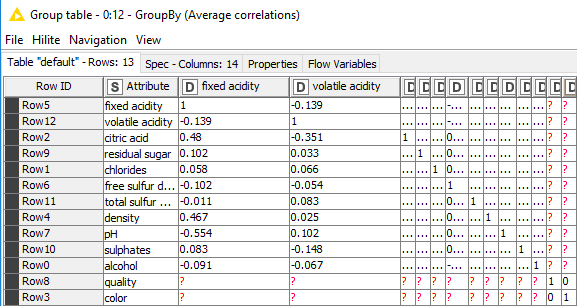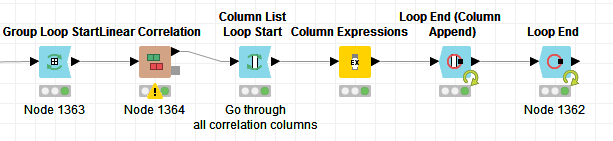# Correlation matrix for each group

Hello all,

I have one single dataset consisting of the same attributes, but the data describes several hundred groups of different data. The data correlates, but respectively only in each group.
Now I would like to generate a correlation matrix for each group of data automatically and then average the output of all correlation matrices into one single correlation matrix.

Any ideas?
If I generate a single correlation matrix out of all the data, there is almost no correlation.

I’m completely new to KNIME, so I’m glad about every hintCheers and thanks, Flo

Hi Florian,

if I understood your problem right, here’s one solution:

Use Group Loop Start node to calculate linear correlation for different groups in the data. In my example workflow, I use data including information on wines, and the group column is color (red/white).

Calculate the linear correlation. Create a new column “Attribute” of the RowIDs because the concatenated table cannot have duplicate RowIDs. The values in the “Attribute” column are the names of the attributes.

Concatenate the results for the different groups with the Loop End node.

Use the GroupBy node to calculate the average correlation between the attributes. In the configuration dialog of the GroupBy node, group column is “Attribute”, aggregation columns are the attrtibutes and aggregation method is mean for all aggregation columns. In the advanced settings in the Manual Aggregation tab, select the column naming option “Keep original name(s)” and check “Retain row order”.

I hope this helps you!

Correlation_example.knwf (128.1 KB)

1 Like

Hi @Maarit,

thanks for this solution but I wonder if you have to remove the self-correlation values - so the ones - before you aggregate the correlation mean-wise to be mathematically really correct.

And how you would do this?

Kind regards

Kiro

Hi Kiro,

I don’t think that the self correlations have to be removed, since we group by an attribute value, and use columns that contain the correlations with other attributes (including the attribute itself) as aggregation columns. This will lead to

1. As many rows (groups) as there are attributes
2. Aggregation of only two values coming from the two groups

The output table of the Loop End node looks like this:

And the ouput table of the GroupBy node looks like this:What do you think?

Regards,
Maarit

Hey @Maarit,
yes you are correct!

Actually I realized that my problem is a bit different.

Nevertheless, assume you would like to aggregate the Matrix even more, so that you have the mean correlation of e.g. ‘fixed acidity’ to all others, now the self-correlation value is disturbing (especially in cases with lots of missing values).

So how you would solve this?

For my own problem I constructed a workflow to get rif of -1, 0, 1 but actually I would like to get rid of values only residing on the self-correlation diagonale.

So something like, assume Matrix A with indices ij; then if i=j set value to NaN.
Do you have any ideas how to solve this?

Kind regards

Kiro

Hi Kiro,

Attached is my suggestion how to solve your problem.

I do the replacement of the self correlations in the following steps:

1. Make attribute values into RowIDs

2. Use the Column List Loop Start node to handle one column at a time

3. Inside the loop, use the Column Expressions node with this code inside:

a = rowId()
b = variable(“currentColumnName”)
if(equals(a,b))
NaN
else
column(variable(“currentColumnName”))

4. Collect all columns back to one table using the Loop End (Column Append) node

Correlation_example2.knwf (137.6 KB)

Regards,
Maarit

2 Likes

Hi @Maarit,

thanks a lot!

This solves the issue!

However, a minor issue remains, since I do not use attribute values in my workflow (I do not want to aggregate the values based on attributes at this stage) I had to loop things:In my case this results in 2129 Iterations (outer loop) each containing a ~ 20X20 Matrix of correlation values which will be processed in the inner loop with your script in the Column Expression node.

It turns out, that this workflow is a bit slow.

Nevertheless, the result is exactly what I want!

Many thanks again!

Kind regards

Kiro

Hello @KiroVandenBerg,

Column Expressions got speedup with KNIME version 4.2.1. so your workflow should be a bit faster if still in useBr,
Ivna